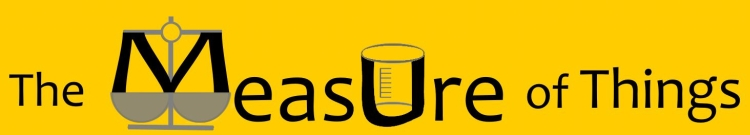Bluebulb Projects presents:Enter a measurement to see comparisons

Equivalents in other units

How big is 325 square feet?

Sort Order:
Closest first | Highest first | Lowest first

 It's about 65,000 times as big as a Quarter. In other words, 325 square feet is 65,330 times the size of a Quarter, and the size of a Quarter is 0.000015310 times that amount. (United States quarter-dollar coin) (a.k.a. Quarter dollar, a.k.a. Washington quarter, a.k.a. two bits)The George Washington quarter, in circulation in various forms since 1932, has a diameter of 24.26 mm (0.955 in) and an area of 0.004975 square feet. It's about 80,000 times as big as a Postage Stamp. In other words, the size of a Postage Stamp is 0.00001000 times 325 square feet. (average; United States dimensions)A typical postage stamp measures an average of 0.004 square feet. One of the most prized stamps among collectors is the US-issued "Inverted Jenny" stamp printed in 1918, which features an upside-down image of Curtiss JN-4 ("Jenny") airplane; a single stamp sold for \$977,500 at a 2007 auction. It's about 85,000 times as big as a Nickel. In other words, 325 square feet is 85,460 times the size of a Nickel, and the size of a Nickel is 0.00001170 times that amount. (United States five-cent coin) (a.k.a. Jefferson nickel)The nickel, in its current design since 1938, has a diameter of 21.21 mm (0.835 in) and an area of 0.003803 square feet. It's about 100,000 times as big as a Penny. In other words, 325 square feet is 106,000 times the size of a Penny, and the size of a Penny is 0.000009430 times that amount. (United States one-cent coin) (a.k.a. Lincoln penny, a.k.a. Cent, a.k.a. One cent piece)The Lincoln penny, in its current design since 1909, has a diameter of 19.05 mm (0.75 in) and an area of 0.00307 square feet. It's about one-one-hundred-thousandth as big as Central Park. In other words, 325 square feet is 0.000008850 times the size of Central Park, and the size of Central Park is 113,000 times that amount. (Manhattan, New York City, New York)The most visited park in the country, Central Park has an area of 36,700,000 square feet. According to a 2005 appraisal, the real estate value of the Park's land is \$528,783,552,000. It's about 100,000 times as big as a Dime. In other words, 325 square feet is 119,900 times the size of a Dime, and the size of a Dime is 0.000008340 times that amount. (United States ten-cent coin) (a.k.a. Roosevelt dime)The dime, in its current design since 1946, has a diameter of 17.91 mm (0.705 in) and an area of 0.002711 square feet. It's about one-two-hundred-thousandth as big as Crawford Ranch. In other words, 325 square feet is 0.0000047130 times the size of Crawford Ranch, and the size of Crawford Ranch is 212,200 times that amount. (a.k.a. Prairie Chapel Ranch, a.k.a. Western White House) (Crawford, Texas)Crawford Ranch, the private estate of President George W. Bush, is a 68,950,000 square feet estate, which, in addition to the house itself and its outbuildings, features seven canyons, three miles of river frontage and a man-made fish pond.